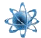xPipeline transport
How do we calculate linepack in pipeline?
Posts  1 - 1  of  1rathorembm
Dear all!
what is procedure to calculate real time line pack of cross country LPG pipeline?We can calculate line fill quantity of pipeline(mass)=volume of pipeline\*density. Density and pressure of pipeline varies with flow rate and temperature of atmosphere.i have little idea about this that first we divide long pipeline in different sections.Measure pressure,density, temperature at all sections(at inlet and outlet of section).use above basic formula. These days all pipelines have real time linepack softwares but when there is OFC cut, and no communication from RTUs.So if someone want to calculate how much material is there in particular section. For example consider any section of pipeline of length"L",flowrate "Q", inlet pressure & density of section is P1 & d1.Outset pressure and density of this section is P2 & d2.How to calculate average density.